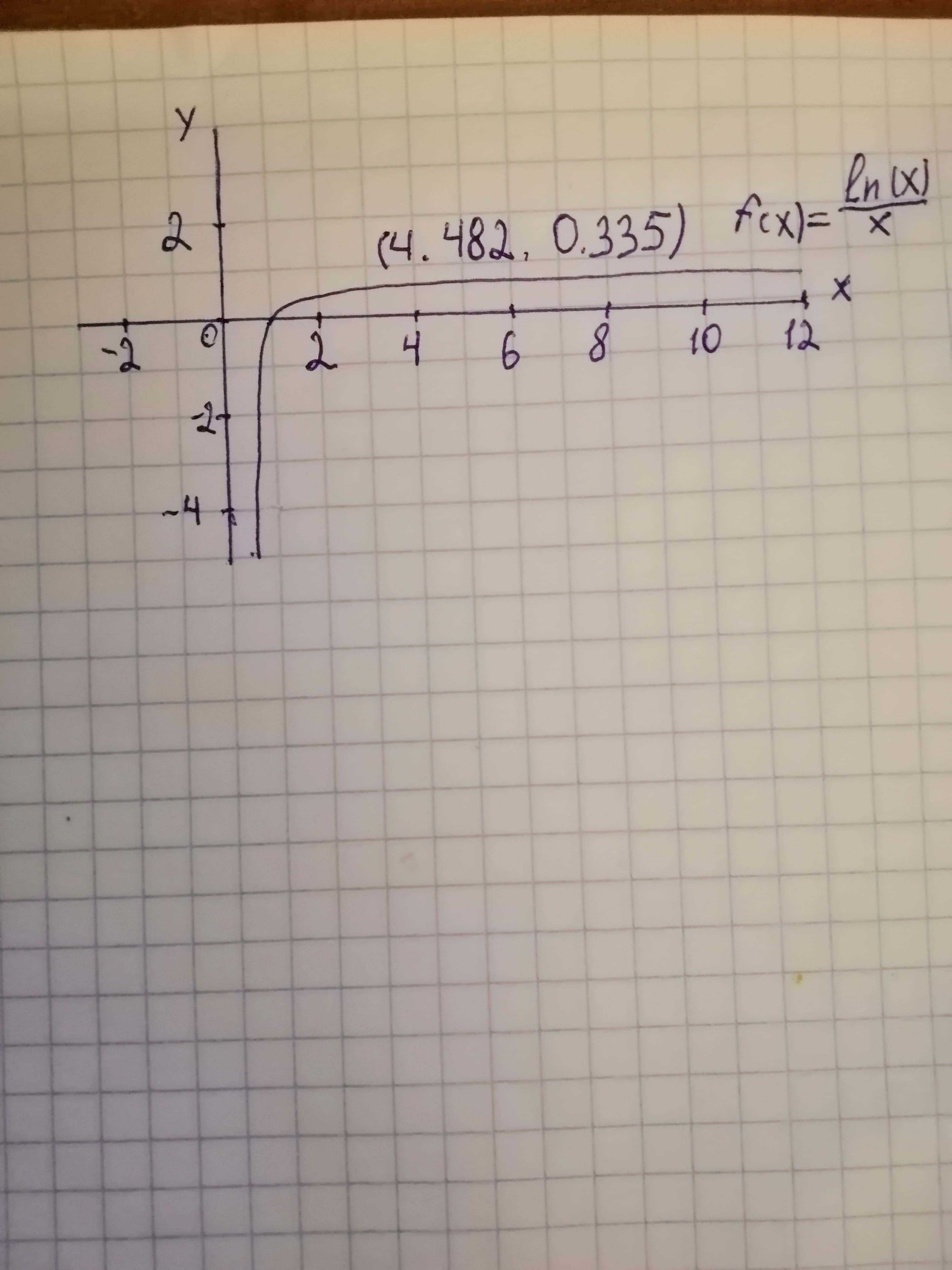Question# Find vert and horz asymptotes, and points of inflection for f(x) = ln(x)/x The graph the function

Confidence intervals
ANSWEREDFind vert and horz asymptotes, and points of inflection for
$$f(x) = \ln(x)/x$$
The graph the function2020-11-27

Step 1
To use :
Vertical asymptotes :
The graph of $$y = f(x)$$ have vertical asymptotes at those value of x for which the denominator is equal to zero.
Horizontal asymptotes:
If the degree (the largest exponent ) of the denominator is bigger than the degree of the numerator , the horizontal asymptotes is the $$x - axis (y = 0).$$
If the degree of the numerator is bigger than the denominator ,there is no horizontal asymptotes.
If the degree of the numerator and denominator are same , the horizontal asymptotes equals the leading coefficient (the coefficient of the largest exponent ) of the numerator divided by the leading coefficient of the denominator.
Inflection point :
An inflection point is a point on the graph at which the the second derivative changes sign.
if $$f'' (x)\ >\ 0$$ then f (x) is concave upwards.
if $$f'' (x)\ <\ 0$$ then f (x) is concave downwards.
Step 2
To find Vertical asymptotes:
Use the definition of vertical asymptotes.
Set the denominator of the given function is zero.
Denominator of the given function is x.
To set the denominator is equal to zero.
To get,
$$x = 0$$
Then, the vertical asymptotes of the given function $$f(x)=\frac{\ln(x)}{x}\ is\ x=0.$$
Step 3
To find the horizontal asymptotes:
To use the above definition of the horizontal asymptotes.
Let $$f(x) = \frac{\ln(x)}{x}$$
Here, the degree(the largest exponent) of the denominator is bigger than the degree of the numerator.To get, the horizontal asymptotes is $$y = 0$$
Then,
The horizontal asymptotes of the function $$f(x) = \frac{\ln(x)}{x}\ is\ y = 0.$$
Step 4
To find the inflection point:
To find the first and second derivative of the given function.
To use the quotient rule.
$$\frac{d}{dx}\left(\frac{u}{v}\right)=\frac{v\ \frac{du}{dx}\ -\ u\ \frac{dv}{dx}}{v^{2}}$$
Then,
$$\frac{d}{dx}\ \left(\frac{\ln(x)}{x}\right)=\frac{x\ \frac{d}{dx}\ln(x)\ -\ \ln(x)\frac{d}{dx}x}{x^{2}}$$
$$f' (x) = \frac{1\ -\ \ln(x)}{x^{2}}$$
Diffrentiate the $$f' (x)$$ again withrespect to x, to get,
$$\frac{d}{dx}\left(\frac{1\ -\ \ln(x)}{x^{2}}\right)=\frac{x^{2}\ \frac{d}{dx}(1\ -\ \ln(x))\ -\ (1\ -\ \ln(x))\ \frac{d}{dx}x^{2}}{x^{4}}$$
$$f'(x) = \frac{-2\ \ln (x)\ +\ 3}{x^{3}}$$
To find the inflection point:
To find where the $$f'' (x)$$ is equal to zero or undefined.
To get,
$$x = e^{\frac{3}{2}},\ x = 0.$$
Next, to identify the inflection point not in the domain or where f (x) is not continuous.
Also, Domain of the function $$f (x) = \frac{\ln(x)}{x}\ is\ x\ >\ 0$$
To get, f(x) is not dfined at $$x = 0$$ and which is not in the domain of the given function.
To get,
$$x = e^{\frac{3}{2}}$$ is the inflection point of the given funtion.
Next, to check the sign of $$f''(x)$$ in the domain of the given funtion.
To use the intervals: $$0\ <\ x\ <\ e^{\frac{3}{2}},\ e^{3}{2}\ <\ x <\ \infty.$$
Let $$f'' (x) = \frac{-2 \ln(x)\ +\ 3}{x^{3}}$$
$$x = e^{\frac{3}{2}}$$
a) $$0\ <\ x\ <\ e^{\frac{3}{2}}$$
Sign: -
Behavior: Concave downward
b) $$x = e^{\frac{3}{2}}$$
Sign: 0
Behavior: Inflection point
c)$$e^{\frac{3}{2}}\ <\ x\ <\ \infty$$
Sign: -
Behavior: Concave upward
Step 5
Next, plug the inflection point $$x = e^{\frac{3}{2}}$$ into the given funcion.
To get,
$$f(x) = \frac{\ln(x)}{x}$$
$$= \frac{\ln (e^{\frac{3}{2}})}{e^{\frac{3}{2}}}$$
$$=\frac{\frac{3}{2}\ln(e)}{e^{\frac{3}{2}}}$$
$$= \frac{3}{2e^{\frac{3}{2}}}$$
To get,
$$f(x) = \frac{3}{2e^{\frac{3}{2}}}$$
That is, $$y = \frac{3}{2e^{\frac{3}{2}}}$$
To get,
$$(x,\ y) =\left(e^{\frac{3}{2}},\ \frac{3}{2e^{\frac{3}{2}}}\right)$$
Therefore,
The inflection point of the $$f(x)=\frac{\ln(x)}{x}\ is\ \left(e^{\frac{3}{2}},\ \frac{3}{2e^{\frac{3}{2}}}\right).$$
Step 6
Graph:..............................